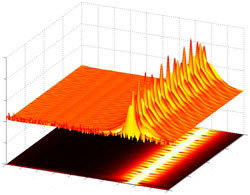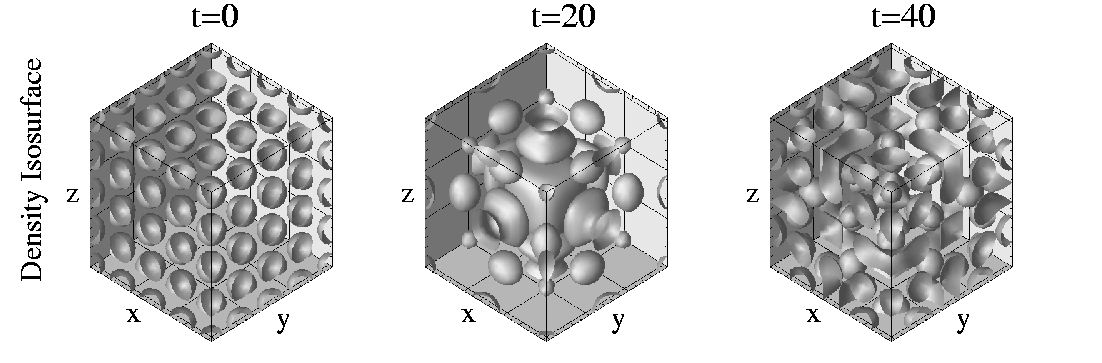# AMATH 581Role
Scientific Computing (MWF 8:30-9:20, Lowe 216)

Lectures and Homework
Video Lectures: Online
Course Notes: amath581 (Book is optional AMAZON)
Discussion Board: Catalyst
Homework: Practice 1 (Due midnight 10/6), HW 1 (Due 10/14), HW 2 (Due 10/28), HW 3 (Due 11/4), HW 4 (Due 11/11), HW 5 (Due 11/22), HW 6 (Due 12/7)
MATLAB: Student Edition (recommended if you do not have access)
SCORELATOR: scorelator (VIDEO DEMO)
Background resources: Beginning Scientific Computing

Instruction
Professor J. Nathan Kutz
Lewis 118
Office Hours: Friday 1pm-3pm Lewis 115 (skype in: TheAmathTA)
-
Teaching Assistant: Meghana Velagar
Office Hours: Monday, Wednesday, Friday 3pm-5pm Lewis 115
EDGE: Monday, Wednesday, Friday 5pm-6pm skype: TheAmathTA

MATLAB on campus
There is MATLAB access at the ICL lab on campus. You can access this also remotely by following the links to "terminal server".

Agenda for Lectures and Notes
9/28 Lecture 1: Notes 1.1, Book 7.1
9/30 Lecture 2: Notes 1.2, Book 7.2
10/3 Lecture 3: Notes 1.3, Book 7.4
10/5 Lecture 4: Notes 1.4, Book 7.5
10/7 Lecture 5: Notes 1.5, Book 7.6
10/10 Lecture 6: Notes bvp4c (notes), Book 7.7
10/12 Lecture 7: Notes 2.1, Book 8.1
10/14 Lecture 8: Notes 2.2, Book 8.2
10/17 Lecture 9: Notes 2.3, Book 8.3
10/19 Lecture 10: Notes 2.4, Book 8.4
10/21 Lecture 11: Notes 2.5, Book 8.5
10/24 Lecture 12: Notes A.1, Book 25.4
10/26 Lecture 13: Notes 3.1, Book 9.1
10/28 Lecture 14: Notes 3.2, Book 9.2
10/31 Lecture 15: Notes 3.3, Book 9.3
11/2 Lecture 16: Notes 3.4, Book 9.4
11/4 Lecture 17: Notes 3.5, Book 9.5
11/7 Lecture 18: Notes 3.6, Book 9.6
11/9 Lecture 19: Notes 4.1, Book 10.1
11/14 Lecture 20: Notes 4.2, Book 10.2
11/16 Lecture 21: Notes 4.3, Book 10.3
11/18 Lecture 22: Notes A.2, Book 25.5
11/21 Lecture 23: Notes 4.4, Book 10.4
11/23 Lecture 24: Notes 4.5, Book 10.5
11/28 Lecture 25: Notes 4.6, Book 10.6
11/30 Lecture 26: Notes 4.7, Book 10.7
12/2 Lecture 27: Notes A.3, Book 25.6
12/5 Lecture 28: Notes 5.1, Book 11.1
12/7 Lecture 29: Notes 5.2, Book 11.2
12/9 Lecture 30: Notes PDEtool (notes), Book 11.3

Prerequisites
Solid background in ODEs and familiarity with PDEs and MATLAB, or permission.
Course Description
Survey of practical numerical solution techniques for ordinary and partial differential equations. Emphasis will be on the implementation of numerical schemes to practical problems of the engineering and physical sciences. Methods for partial differential equations will include finite difference, finite element and spectral techniques. Full use will be made of MATLAB and its built in programming and solving functionality.
Objectives

How to recognize and solve numerically practical problems which may arise in your research. We will solve some serious problems using the full power of MATLAB's built in functions and routines. This class is geared for those who need to get the basics in scientific computing. All major types of PDEs (parabolic, elliptic, and hyperbolic) will be considered in 1D, 2D and 3D in problems ranging from quantum mechanics to fluid flows.

NOTE: This course is a survey of computational methods. The focus is on the implemention of numerical schemes with significant aid from built-in MATLAB functionality such as FFTs, fast matrix solvers, etc. It is not a course in numerical analysis since our coverage of many technical issues is only cursory. A much more comprehensive and detailed treatment of some of the methods covered here is given in AMATH 584, 585, 586.#### Figure

Dynamics of a repulsive Bose-Einstein condensate trapped in a 3-D lattice potential. The equation was solved using a filtered spectral method in space and 4th-order Runge-Kutta in time. By the end of this course, you should be able to perform this numerical simulation.

## Textbook & Notes

There will be no text for this course. I will provide my notes on-line for you to download. I have several texts which will be on reserve at the library to look through the different sections.
Lecture Notes: .pdf

Reference Texts on Reserve:

1. R. L. Burden and J. D. Faires, Numerical Analysis (Sixth Edition). Brooks/Cole, 1997.
2. L. N. Trefethen, Finite Difference and Spectral Methods. (freely available).
3. L. N. Trefethen, Spectral Methods in MATLAB. SIAM.
4. L. N. Trefethen and D. Bau, Numerical Linear Algebra. SIAM.
5. J. C. Strikwerda, Finite Difference Schemes and Partial Differential Equations. CRC Press.
6. D. R. Durran, Numerical Methods for Wave Equations in Geophysical Fluid Dynamics. Springer.

## Syllabus

(1) Solution Methods for Differential Equations: (2 weeks)

We will begin with ODE solvers applied to both initial and boundary value problems. Our application will be to finding the eigenstates of a quantum mechanical problem or of an optical waveguide.

(a) Initial value problems
(b) Euler method, 2nd- and 4th-order Runge-Kutta, Adams-Bashford
(c) Stability and time stepping issues
(d) Boundary values problems: shooting/collocation/relaxation

(2) Finite Difference Schemes for Partial Differential Equations: (3 weeks)

We will introduce the idea of finite-differencing of differential operators. Our application will be to two problems: vibrating modes of a drum and the evolution of potential vorticity in an advection-diffusion problem of fluid mechanics.

(a) Collocation
(b) Stability and CFL conditions
(c) Time and space stepping routines
(d) Tri-diagonal matrix operations

(3) Spectral Methods for Partial Differential Equations: (3 weeks)

Transform methods for PDEs will be introduced with special emphasis given to the Fast-Fourier Transform. We will revisit the potential vorticity in an advection-diffusion problem of fluid mechanics by using these spectral techniques.

(a) The Fast-Fourier transform (FFT)
(b) Chebychev transforms
(c) Time and space stepping routines
(d) Numerical filtering algorithms

(4) Finite Element Schemes for Partial Differential Equations: (2 weeks)

For complicated computational domains, the use of a finite element scheme is compulsory. The steady-state flow of a fluid over various airfoils will be considered.

(a) Mesh generation